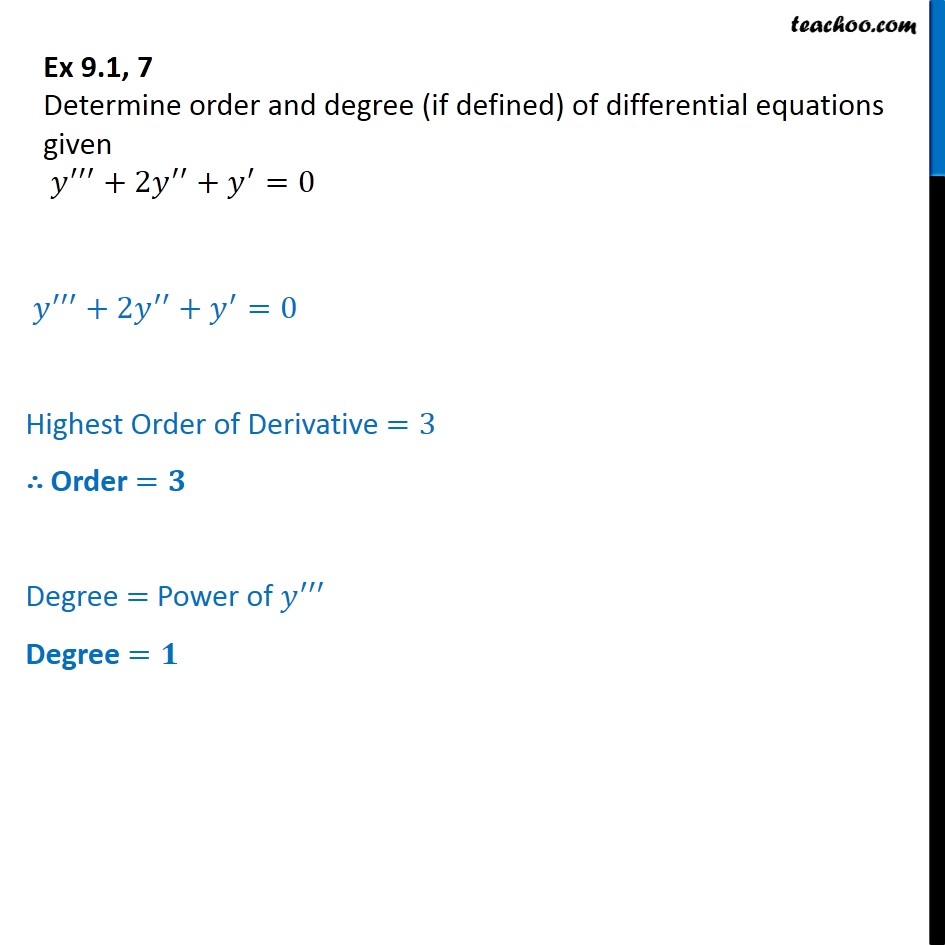Order and Degree

Chapter 9 Class 12 Differential Equations
Concept wiseLearn in your speed, with individual attention - Teachoo Maths 1-on-1 Class

### Transcript

Ex 9.1, 7 Determine order and degree (if defined) of differential equations given y +2y + y =0 y +2y + y =0 Highest Order of Derivative =3 Order = Degree = Power of y Degree =